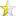Mar 13, 2011 (last update: Mar 13, 2011)

# Simple XOR encryptionAlright, beginner's encryption lesson:

everything on a computer is stored as binary data, in the form of bytes (8 bits, or individual 1's or 0's)

Binary data can easily be "encrypted" with a "key" based on a little boolean operation called an xor, or exclusive or.
when we xor a single bit (a 1 or 0) with another bit:

if 1 bit is true, and 1 bit is false, it returns true, otherwise it returns false;
sooo:

1 xor 1 = 0
1 xor 0 = 1
0 xor 1 = 1
0 xor 0 = 0

now one reason this is useful is if we take the new bit and xor it with the same key (the second bit) the result will always be the first bit.
so:

1 xor 1 xor 1 = 1;
1 xor 0 xor 0 = 1;

Of course on a single bit, it wouldn't do much good, but when you get into higher levels of memory it's a fairly simple and (sometimes) effective (see later in article) encryption method.

Now when I say everything is stored as binary data, that also means strings, a single char is stored as either ascii or unicode (or some other protocol, but those are the only two I've seen) values.

for example:

The letter 'h' in ascii = 104
The letter 'i' in ascii = 105

converting from an integer to a binary number is a little difficult, but doable.
when you look at a binary number, you can read it in reverse and add the value of each bit, which is as follows

2x 25 24 23 22 21 20
so the binary number
01110011

 ``12`` ``````0 1 1 1 0 0 1 1 0 + 64 + 32 + 16 + 0 + 0 + 2 + 1``````

is 115 or 's'

now that's all well and good, you can send little notes to your friends, and no one can tell what the heck your saying, but of course you could just write the binary stuff, and it would perform the same action =)

In c++ the ^ operator is the xor operator.
if I have a string, that I want to encrypt, I can do something like this:

 ``12345678910`` `````` string toEncrypt = "fail"; char keyToEncrypt = 's'; //remember 115 in ascii for (int temp = 0; temp < toEncrypt.size(); temp++) toEncrypt[temp] ^= keyToEncrypt; cout << "nThe encrypted data = " << toEncrypt; for (int temp = 0; temp < toEncrypt.size(); temp++) toEncrypt[temp] ^= keyToEncrypt; //notice we're using the exact same key, to unencrypt the data. cout << "nThe unencrypted data = " << toEncrypt;``````

Of course this is all well and good, but here's an excersize for anyone who's using a simple key for their data, if someone has access to your program. And they can encrypt some data of their own, and have access to the output of that encryption, they can get your key. Example:

 ``12345678910`` `````` char original = "f"; char key = "s"; char end; char getKey; end = original ^ key; // now here's the kicker getKey = original ^ end;``````

So you have to get tricky, one reeeeeaaaaly easy way to encrypt and decrypt the data a little more securely, could be something like this:

 ``123456789101112131415`` `````` string original = "super flying monkeys are aweseome, aren't they?"; cout << "Original data = " << original; string encrypted = ""; string unencrypt = ""; char key = 'x'; for (int temp = 0; temp < original.size(); temp++){ encrypted += original[temp] ^ (int(key) + temp) % 255; } cout << "nEncrypted data = " << encrypted; for (int temp = 0; temp < original.size(); temp++){ unencrypted += encrypted[temp] ^ (int(key) + temp) % 255; } cout << "nUnencrypted data = " << unencrypt;``````

Of course, you can should do something other than simple incrementing, because that's pretty easy to sniff.

But there you have it, a simple encryption lesson!